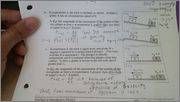# Momentum conservation

In the pictures

Fnet = dp/dt

## The Attempt at a Solution

I know in part b there is a net external force (gravity) that does positive work, fnet > 0, thus dp/dt is positive. Does the spring in part c increase or decrease the momentum of the system? I am not too sure ofWhat is 'the system?' Is it both blocks or one block? Do you see how the answer is different in either case?What is 'the system?' Is it both blocks or one block? Do you see how the answer is different in either case?
The system consists of the two gliders. So it appears that the spring is indeed an external force. But the direction the spring accelerates the block is in negative direction, so I am not sure how the TOTAL momentum of the system will change.

haruspex